## Getting started

The function analyze_objects() can be used to count objects in an image. Let us start with a simple example with the image object_300dpi.png available on the GitHub page. To facilitate the image importation from this folder, a helper function image_pliman() is used.

library(pliman)
#> The legacy packages maptools, rgdal, and rgeos, underpinning the sp package,
#> which was just loaded, will retire in October 2023.
#> Please refer to R-spatial evolution reports for details, especially
#> https://r-spatial.org/r/2023/05/15/evolution4.html.
#> It may be desirable to make the sf package available;
#> package maintainers should consider adding sf to Suggests:.
#> The sp package is now running under evolution status 2
#>      (status 2 uses the sf package in place of rgdal)
#> |==========================================================|
#> | Tools for Plant Image Analysis (pliman 2.0.1)            |
#> | Author: Tiago Olivoto                                    |
#> | Type citation('pliman') to know how to cite pliman     |
#> | Visit 'http://bit.ly/pkg_pliman' for a complete tutorial |
#> |==========================================================|
img <- image_pliman("objects_300dpi.jpg", plot = TRUE)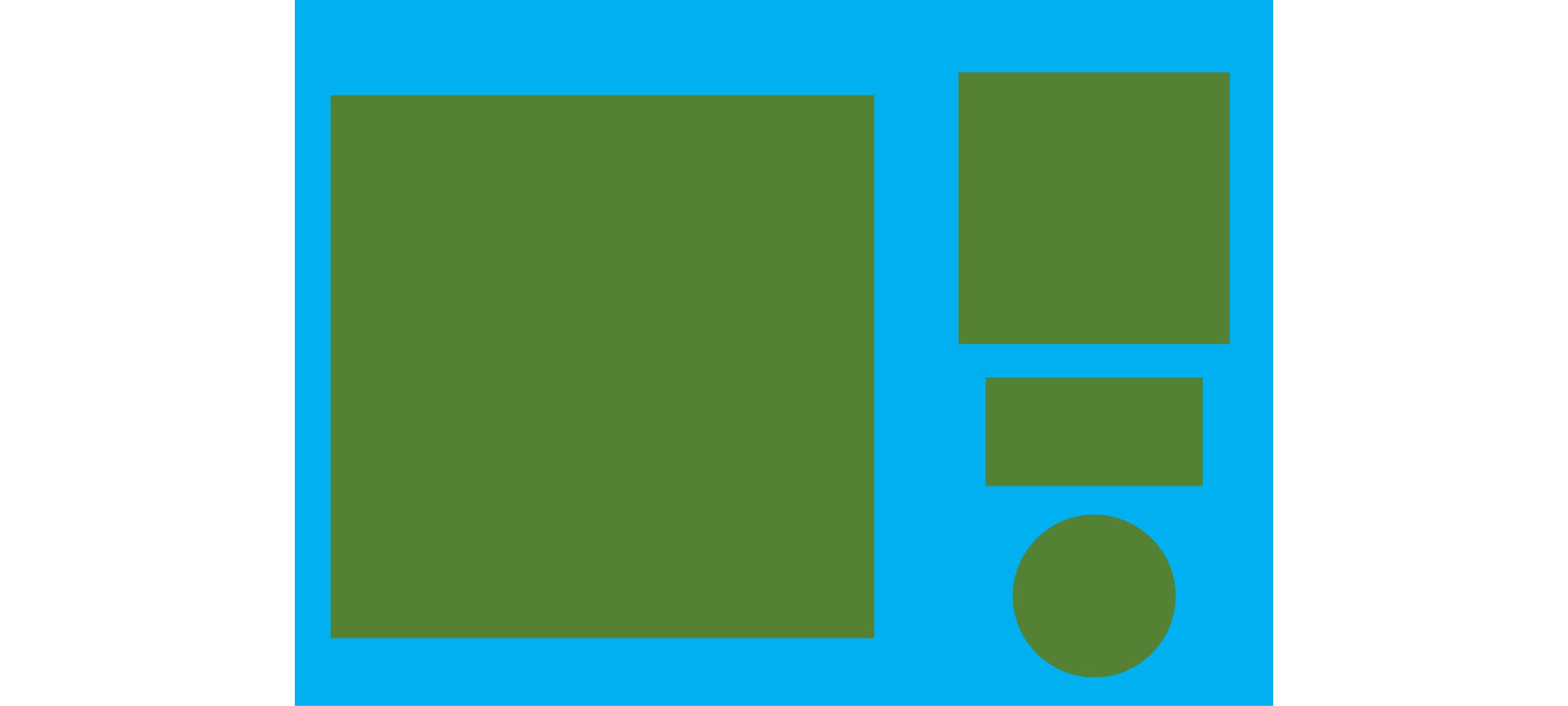The above image was produced with Microsoft PowerPoint. It has a known resolution of 300 dpi (dots per inch) and shows four objects

• Larger square: 10 x 10 cm (100 cm2)
• Smaller square: 5 x 5 cm (25 cm2)
• Rectangle: 4 x 2 cm (8 cm2)
• Circle: 3 cm in diameter (~7.08 cm2)

To count the objects in the image we use analyze_objects() and inform the image object (the only mandatory argument). First, we use image_binary() to see the most suitable index to segment the objects from the background. By default, the R, G, B (first row) and their normalized values (second row) are used.

image_binary(img)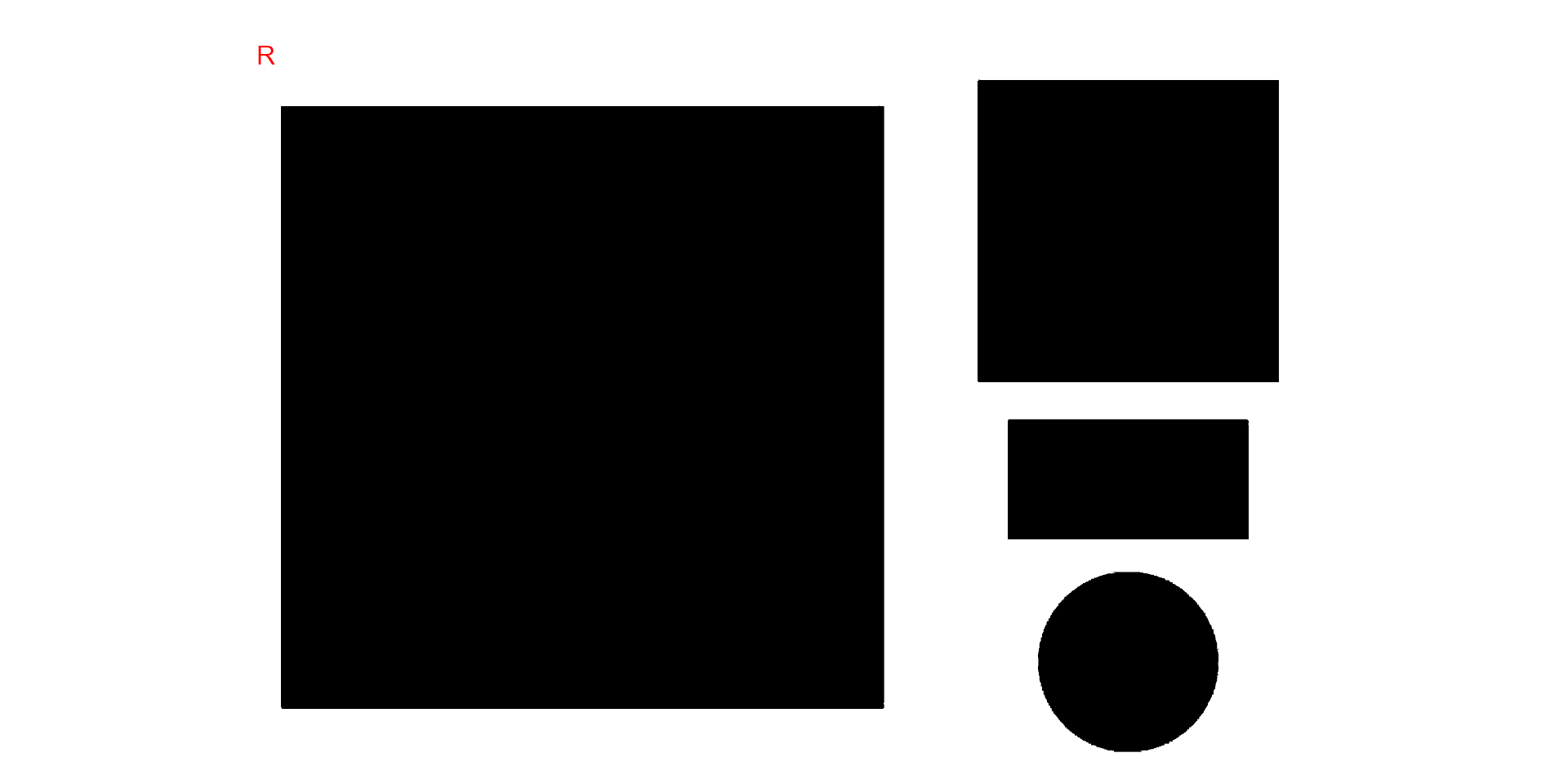## Analyzing objects

img_res <-
analyze_objects(img,
marker = "id",
index = "B") # use blue index to segment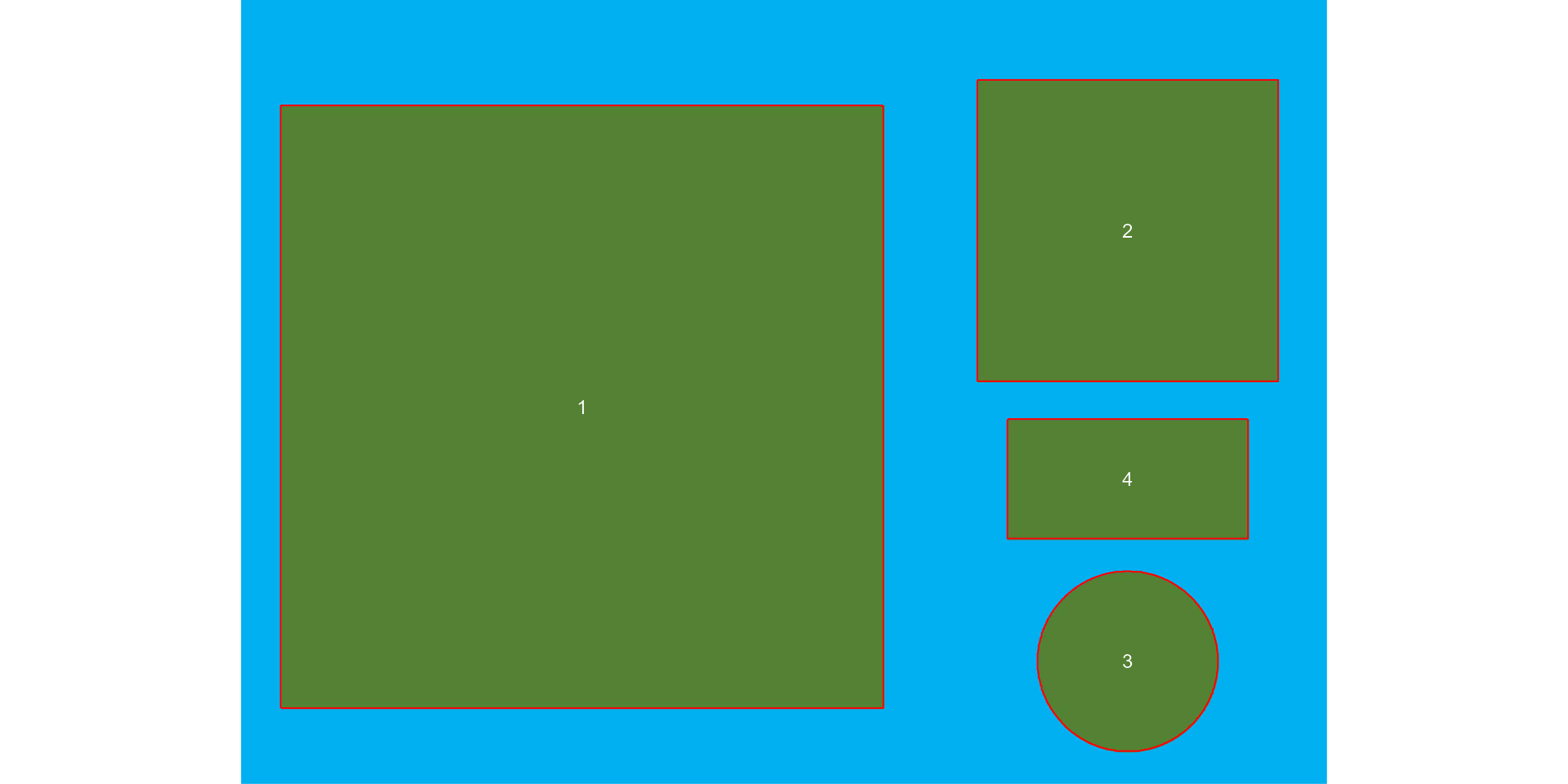The results were stored in img_res. Since there is no scale declared in the above example, we have no idea about the actual area of the objects in cm2, only in pixels. In this case, we use get_measures() to adjust the measures from pixels to metric units.

There are two main ways of adjusting the object measures (from pixels to cm, for example). The first one is to declare the known area, perimeter, or radius of a given object. The measure for the other objects will be then computed by a simple rule of three. The second one is by declaring a known image resolution in dpi (dots per inch). In this case, the perimeter, area, and radius will be adjusted by the informed dpi.

### Declaring a known value

Since we have known the area of the larger square (object 1), let us adjust the area of the other objects in the image using that.

get_measures(img_res,
id = 1,
area ~ 100) |>
str()
#> -----------------------------------------
#> measures corrected with:
#> object id: 1
#> area     : 100
#> -----------------------------------------
#> Total    : 40.001
#> Average  : 13.334
#> -----------------------------------------
#> Classes 'measures' and 'data.frame': 3 obs. of  34 variables:
#>  $id : num 2 3 4 #>$ x                   : num  1737 1737 1736
#>  $y : num 452 1295 938 #>$ area                : num  25 7.05 7.95
#>  $area_ch : num 24.92 7.05 7.9 #>$ perimeter           : num  19.9 10.1 11.9
#>  $radius_mean : num 2.86 1.49 1.67 #>$ radius_min          : num  2.492 1.482 0.988
#>  $radius_max : num 3.53 1.51 2.23 #>$ radius_sd           : num  0.31434 0.00396 0.42388
#>  $diam_mean : num 5.73 2.99 3.34 #>$ diam_min            : num  4.98 2.96 1.98
#>  $diam_max : num 7.06 3.02 4.45 #>$ major_axis          : num  2.04 1.06 1.48
#>  $minor_axis : num 2.036 1.053 0.874 #>$ caliper             : num  7.01 3 4.43
#>  $length : num 5 3 3.99 #>$ width               : num  4.99 3 1.98
#>  $radius_ratio : num 1.42 1.02 2.25 #>$ theta               : num  -1.57 0.459 0
#>  $eccentricity : num 0.0505 0.1114 0.808 #>$ form_factor         : num  0.79 0.873 0.704
#>  $narrow_factor : num 1.4 1 1.11 #>$ asp_ratio           : num  1 1 2.01
#>  $rectangularity : num 0.998 1.278 0.994 #>$ pd_ratio            : num  2.85 3.36 2.69
#>  $plw_ratio : num 2 1.68 2 #>$ solidity            : num  1 1 1.01
#>  $convexity : num 0.75 0.909 0.836 #>$ elongation          : num  0.00169 0.00113 0.50318
#>  $circularity : num 15.9 14.4 17.8 #>$ circularity_haralick: num  9.11 377.4 3.94
#>  $circularity_norm : num 0.787 0.868 0.7 #>$ coverage            : num  0.1068 0.0301 0.034

The same can be used to adjust the measures based on the perimeter or radius. Let us adjust the perimeter of objects by the perimeter of object 2 (20 cm).

get_measures(img_res,
id = 2,
perimeter ~ 20) |>
str()
#> -----------------------------------------
#> measures corrected with:
#> object id: 2
#> perimeter     : 20
#> -----------------------------------------
#> Total    : 62.081
#> Average  : 20.694
#> -----------------------------------------
#> Classes 'measures' and 'data.frame': 3 obs. of  34 variables:
#>  $id : num 1 3 4 #>$ x                   : num  668 1737 1736
#>  $y : num 797 1295 938 #>$ area                : num  100.52 7.09 7.99
#>  $area_ch : num 100.35 7.09 7.94 #>$ perimeter           : num  40 10.1 11.9
#>  $radius_mean : num 5.75 1.5 1.67 #>$ radius_min          : num  5.01 1.49 0.99
#>  $radius_max : num 7.08 1.51 2.23 #>$ radius_sd           : num  0.63056 0.00397 0.42499
#>  $diam_mean : num 11.5 3 3.35 #>$ diam_min            : num  10.02 2.97 1.98
#>  $diam_max : num 14.16 3.03 4.46 #>$ major_axis          : num  4.09 1.06 1.49
#>  $minor_axis : num 4.088 1.056 0.876 #>$ caliper             : num  14.08 3.01 4.45
#>  $length : num 14.16 3.01 4 #>$ width               : num  14.15 3.01 1.99
#>  $radius_ratio : num 1.41 1.02 2.25 #>$ theta               : num  0.783 0.459 0
#>  $eccentricity : num 0.0253 0.1114 0.808 #>$ form_factor         : num  0.788 0.873 0.704
#>  $narrow_factor : num 0.995 1 1.112 #>$ asp_ratio           : num  1 1 2.01
#>  $rectangularity : num 1.992 1.278 0.994 #>$ pd_ratio            : num  2.84 3.36 2.69
#>  $plw_ratio : num 1.41 1.68 2 #>$ solidity            : num  1 1 1.01
#>  $convexity : num 0.75 0.909 0.836 #>$ elongation          : num  0.00043 0.00113 0.50318
#>  $circularity : num 15.9 14.4 17.8 #>$ circularity_haralick: num  9.12 377.4 3.94
#>  $circularity_norm : num 0.787 0.868 0.7 #>$ coverage            : num  0.4274 0.0301 0.034

### Declaring the image resolution

If the image resolution is known, all the measures will be adjusted according to this resolution. Let us to see a numerical example with pixels_to_cm(). This function converts the number of pixels ($$px$$) to cm, considering the image resolution in $$dpi$$, as follows: $$cm = px \times (2.54/dpi)$$. Since we know the number of pixels of the larger square, its perimeter in cm is given by

# number of pixels for the highest square perimeter
ls_px <- img_res$results$perimeter
pixels_to_cm(px = ls_px, dpi = 300)
#>  39.9294

The perimeter of object 1 adjusted by the image resolution is very close to the true (40 cm). Bellow, the values of all measures are adjusted by declaring the dpi argument in get_measures().

get_measures(img_res, dpi = 300) |> str()
#> Classes 'measures' and 'data.frame': 4 obs. of  34 variables:
#>  $id : num 1 2 3 4 #>$ x                   : num  668 1737 1737 1736
#>  $y : num 797 452 1295 938 #>$ area                : num  99.98 25 7.05 7.95
#>  $area_ch : num 99.81 24.91 7.05 7.9 #>$ perimeter           : num  39.9 19.9 10.1 11.9
#>  $radius_mean : num 5.73 2.86 1.49 1.67 #>$ radius_min          : num  4.994 2.491 1.482 0.988
#>  $radius_max : num 7.06 3.53 1.51 2.23 #>$ radius_sd           : num  0.62885 0.31432 0.00396 0.42384
#>  $diam_mean : num 11.46 5.73 2.99 3.34 #>$ diam_min            : num  9.99 4.98 2.96 1.98
#>  $diam_max : num 14.12 7.06 3.02 4.45 #>$ major_axis          : num  4.08 2.04 1.06 1.48
#>  $minor_axis : num 4.077 2.036 1.053 0.874 #>$ caliper             : num  14.05 7.01 3 4.43
#>  $length : num 14.12 5 3 3.99 #>$ width               : num  14.11 4.99 3 1.98
#>  $radius_ratio : num 1.41 1.42 1.02 2.25 #>$ theta               : num  0.783 -1.57 0.459 0
#>  $eccentricity : num 0.0253 0.0505 0.1114 0.808 #>$ form_factor         : num  0.788 0.79 0.873 0.704
#>  $narrow_factor : num 0.995 1.402 1 1.112 #>$ asp_ratio           : num  1 1 1 2.01
#>  $rectangularity : num 1.992 0.998 1.278 0.994 #>$ pd_ratio            : num  2.84 2.85 3.36 2.69
#>  $plw_ratio : num 1.41 2 1.68 2 #>$ solidity            : num  1 1 1 1.01
#>  $convexity : num 0.75 0.75 0.909 0.836 #>$ elongation          : num  0.00043 0.00169 0.00113 0.50318
#>  $circularity : num 15.9 15.9 14.4 17.8 #>$ circularity_haralick: num  9.12 9.11 377.4 3.94
#>  $circularity_norm : num 0.787 0.787 0.868 0.7 #>$ coverage            : num  0.4274 0.1068 0.0301 0.034

## Counting crop grains

Here, we will count the grains in the image soybean_touch.jpg. This image has a cyan background and contains 30 soybean grains that touch with each other. Two segmentation strategies are used. The first one is by using is image segmentation based on color indexes.

soy <-        image_pliman("soybean_touch.jpg")
grain <-      image_pliman("soybean_grain.jpg")
background <- image_pliman("la_back.jpg")
image_combine(soy, grain, background, ncol = 3)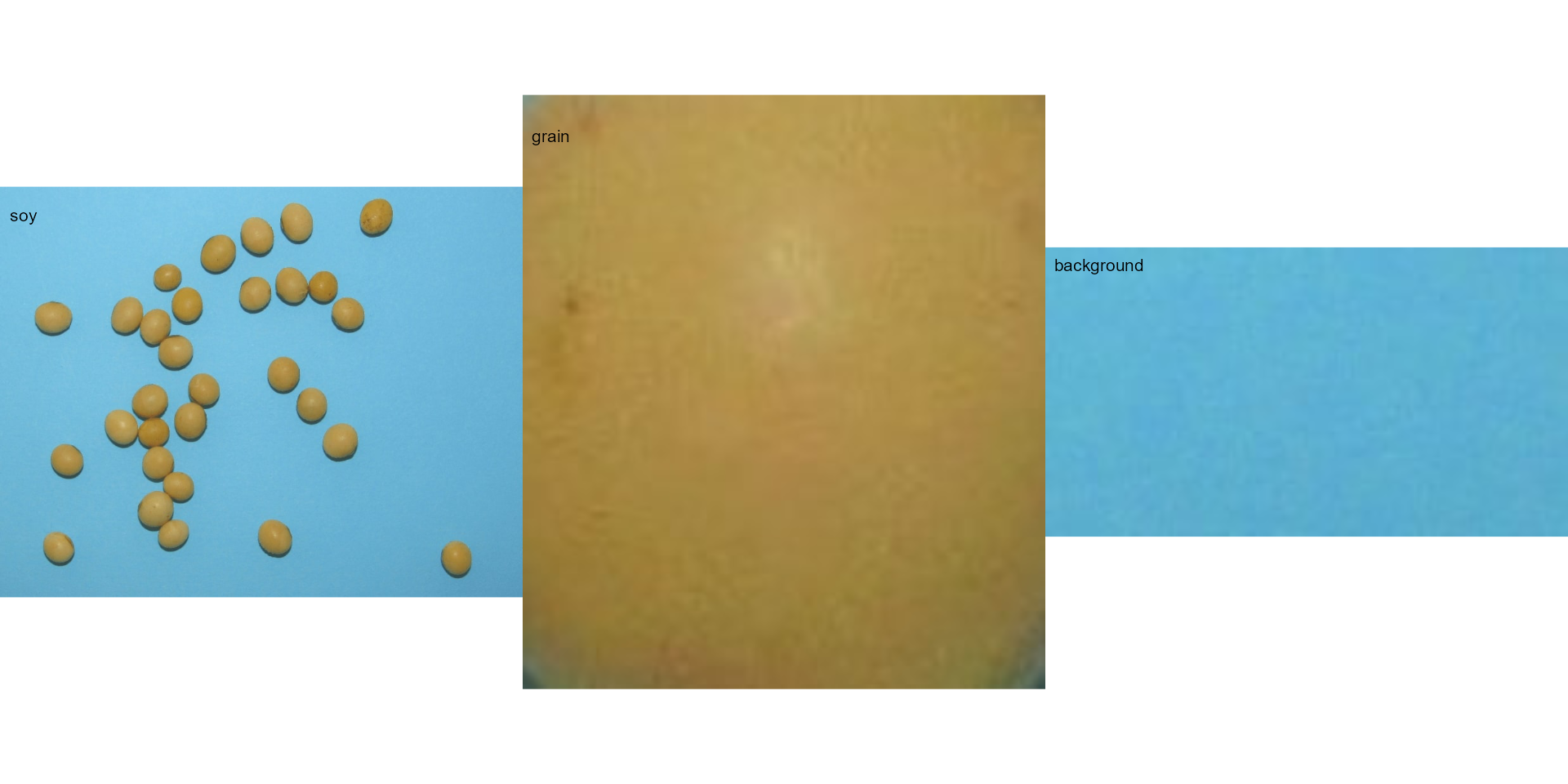The function analyze_objects() segment the image using as default the normalized blue index, as follows $$NB = (B/(R+G+B))$$, where $$R$$, $$G$$, and $$B$$ are the red, green, and blue bands. Objects are count and the segmented objects are colored with random permutations.

count2 <-
analyze_objects(soy,
index = "NB") # default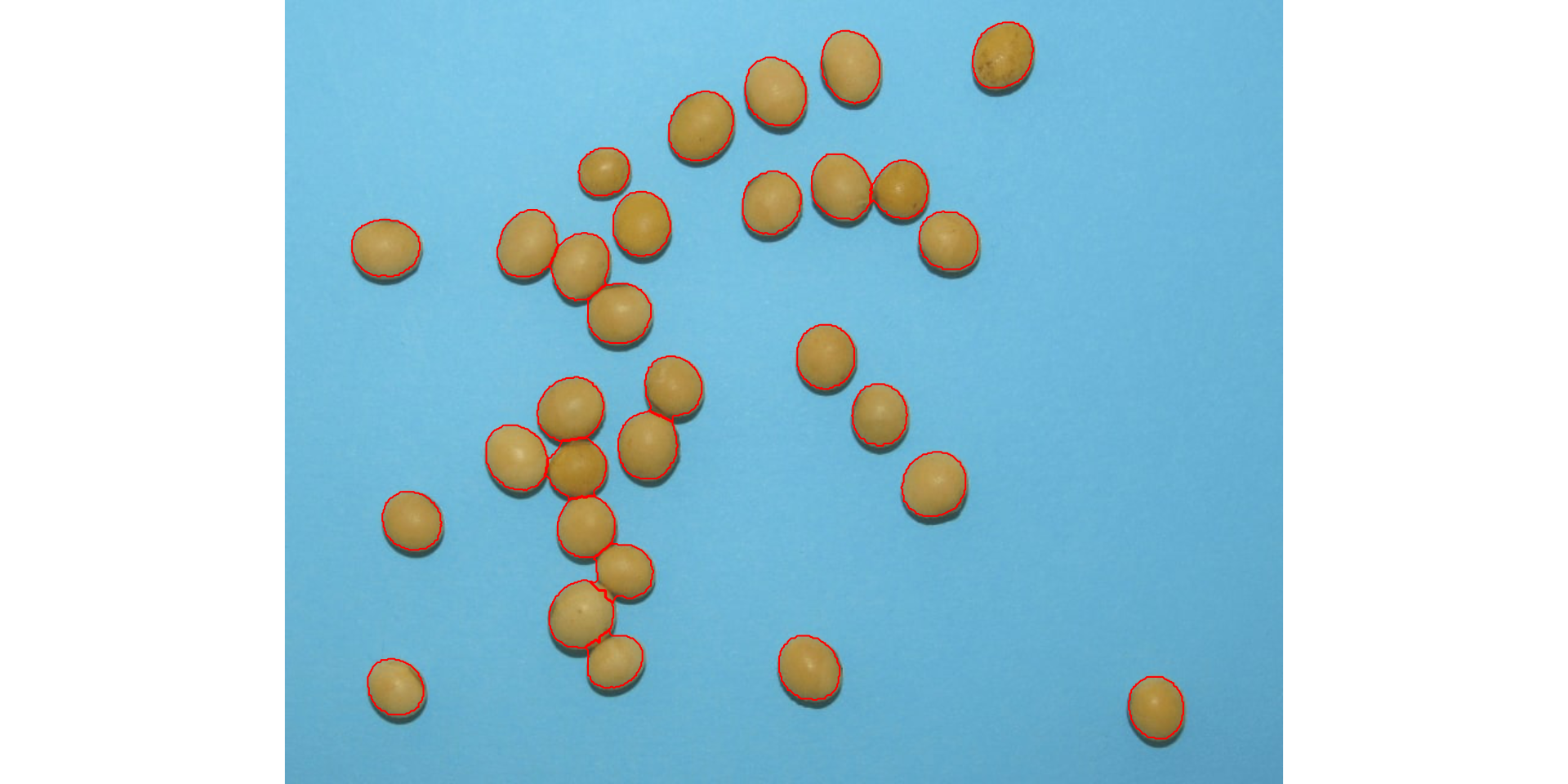Users can set show_contour = FALSE to remove the contour line and identify the objects (in this example the grains) by using the arguments marker = "id". The color of the background can also be changed with col_background.

count <-
analyze_objects(soy,
show_contour = FALSE,
marker = "id",
show_segmentation = FALSE,
col_background = "white",
index = "NB") # default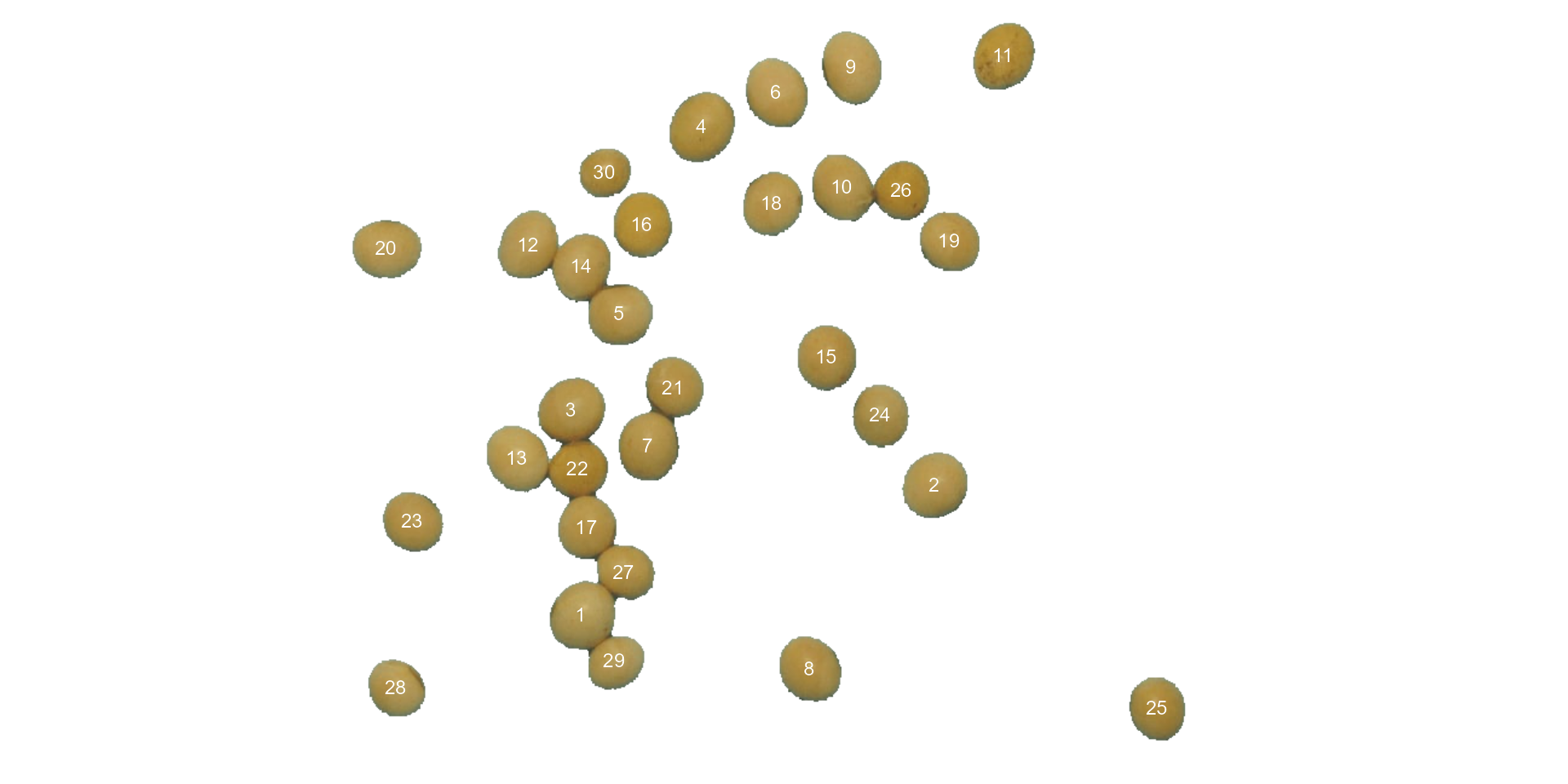# Get the object measures
measures <- get_measures(count)
str(measures)
#> Classes 'measures' and 'data.frame': 30 obs. of  34 variables:
#>  $id : num 1 2 3 4 5 6 7 8 9 10 ... #>$ x                   : num  245 537 237 344 277 ...
#>  $y : num 509 401 339 105 260 ... #>$ area                : num  2279 2289 2310 2436 2159 ...
#>  $area_ch : num 2304 2262 2288 2408 2122 ... #>$ perimeter           : num  184 178 181 186 172 ...
#>  $radius_mean : num 26.5 26.6 26.7 27.5 25.8 ... #>$ radius_min          : num  23 24.8 24 24.3 24.2 ...
#>  $radius_max : num 29.4 28.7 29.4 30.5 28 ... #>$ radius_sd           : num  1.375 0.966 1.238 1.74 0.801 ...
#>  $diam_mean : num 53 53.1 53.4 54.9 51.5 ... #>$ diam_min            : num  45.9 49.7 48 48.6 48.5 ...
#>  $diam_max : num 58.8 57.4 58.9 61.1 56.1 ... #>$ major_axis          : num  19.3 19.5 19.8 20.8 18.7 ...
#>  $minor_axis : num 18.2 18 17.9 18 17.7 ... #>$ caliper             : num  57.3 56.9 57.7 61 54.4 ...
#>  $length : num 56.6 56.5 57.2 61 54 ... #>$ width               : num  51.5 52.4 52 51 50.5 ...
#>  $radius_ratio : num 1.28 1.16 1.23 1.26 1.16 ... #>$ theta               : num  -0.828 -0.804 -0.637 -0.979 -0.217 ...
#>  $eccentricity : num 0.328 0.387 0.428 0.495 0.325 ... #>$ form_factor         : num  0.85 0.906 0.886 0.889 0.92 ...
#>  $narrow_factor : num 1.01 1.01 1.01 1 1.01 ... #>$ asp_ratio           : num  1.1 1.08 1.1 1.2 1.07 ...
#>  $rectangularity : num 1.28 1.29 1.29 1.28 1.26 ... #>$ pd_ratio            : num  3.2 3.13 3.14 3.04 3.16 ...
#>  $plw_ratio : num 1.7 1.64 1.66 1.66 1.64 ... #>$ solidity            : num  0.989 1.012 1.009 1.012 1.017 ...
#>  $convexity : num 0.887 0.879 0.911 0.919 0.898 ... #>$ elongation          : num  0.089 0.0737 0.0911 0.1639 0.0643 ...
#>  $circularity : num 14.8 13.9 14.2 14.1 13.7 ... #>$ circularity_haralick: num  19.3 27.5 21.6 15.8 32.2 ...
#>  $circularity_norm : num 0.821 0.875 0.855 0.858 0.887 ... #>$ coverage            : num  0.00426 0.00428 0.00432 0.00456 0.00404 0.0043 0.00414 0.00406 0.00432 0.00405 ...

In the following example, we will select objects with an area above the average of all objects by using lower_size = 2057.36. Additionally, we will use the argument show_original = FALSE to show the results as colors (non-original image).

analyze_objects(soy,
marker = "id",
show_original = FALSE,
lower_size = 2057.36,
index = "NB") # default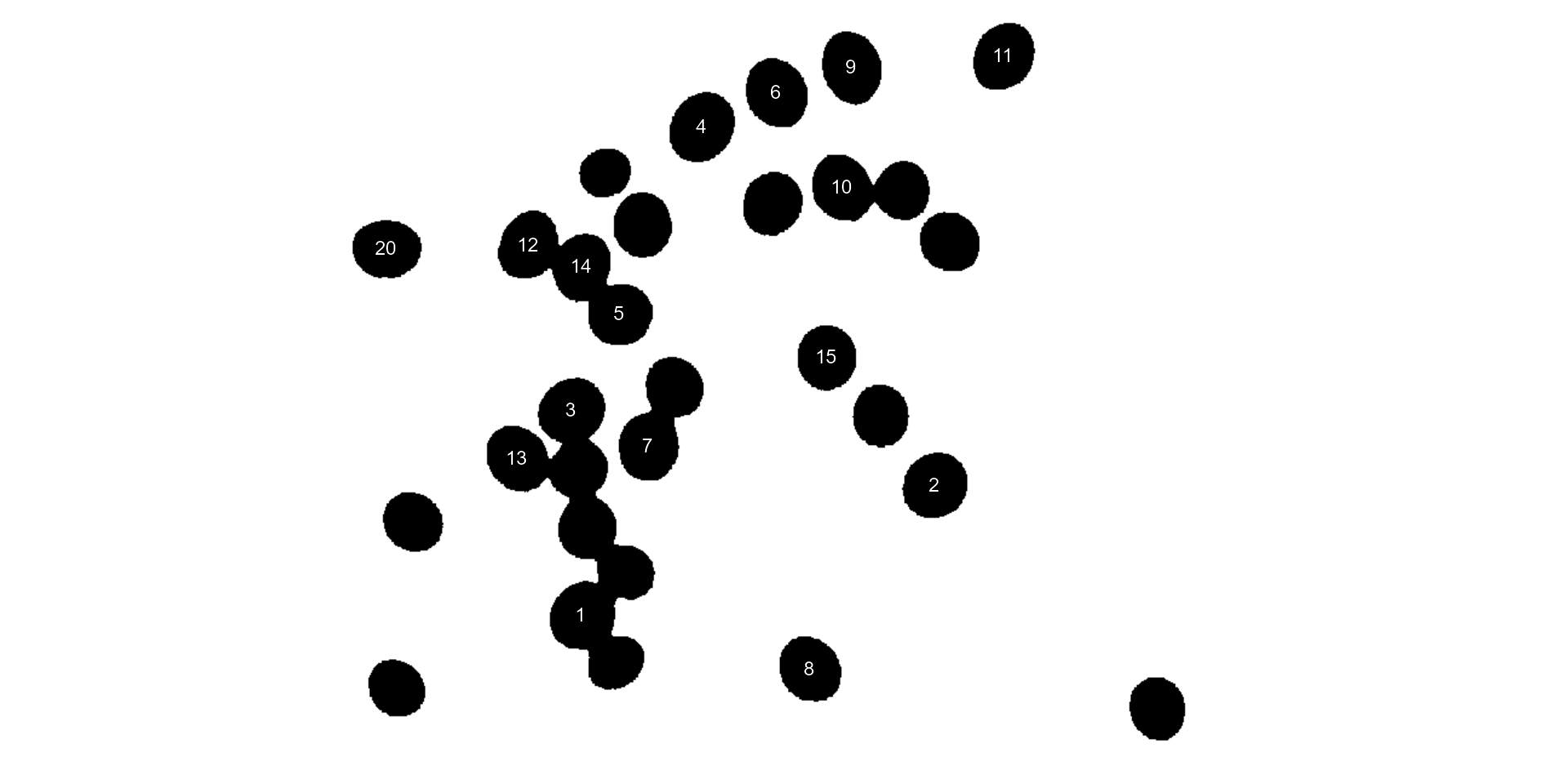Users can also use the topn_* arguments to select the top n objects based on either smaller or largest areas. Let’s see how to point out the 5 grains with the smallest area, showing the original grains in a blue background. We will also use the argument index to choose a personalized index to segment the image. Just for comparison, we will set up explicitly the normalized blue index by calling index = "B/(R+G+B)".

analyze_objects(soy,
marker = "id",
topn_lower = 5,
col_background = "blue",
index = "B/(R+G+B)") # default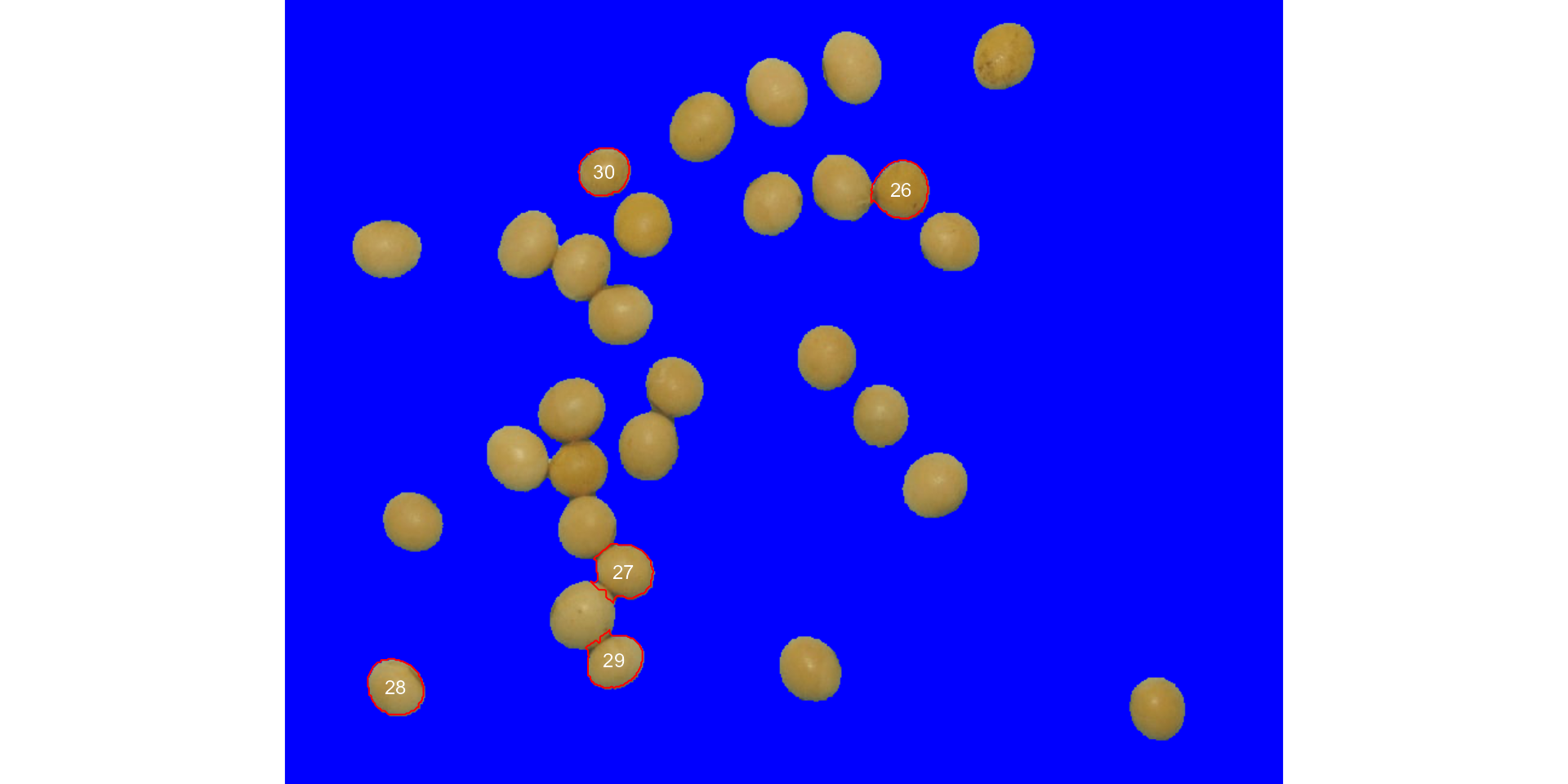## Using sample palettes

Sometimes it is difficult to choose an image index that segments the image efficiently (even using index ). In pliman users have an alternative image segmentation strategy that is using sample color palettes. In this case, users can say to analyze_objects which color palettes are to be used for background and foreground. A generalized linear model (binomial family) is then used to predict the value of each pixel (background or foreground). Let’s see how the grains of the above image can be counted with this strategy.

analyze_objects(img = soy,
background = background,
foreground = grain)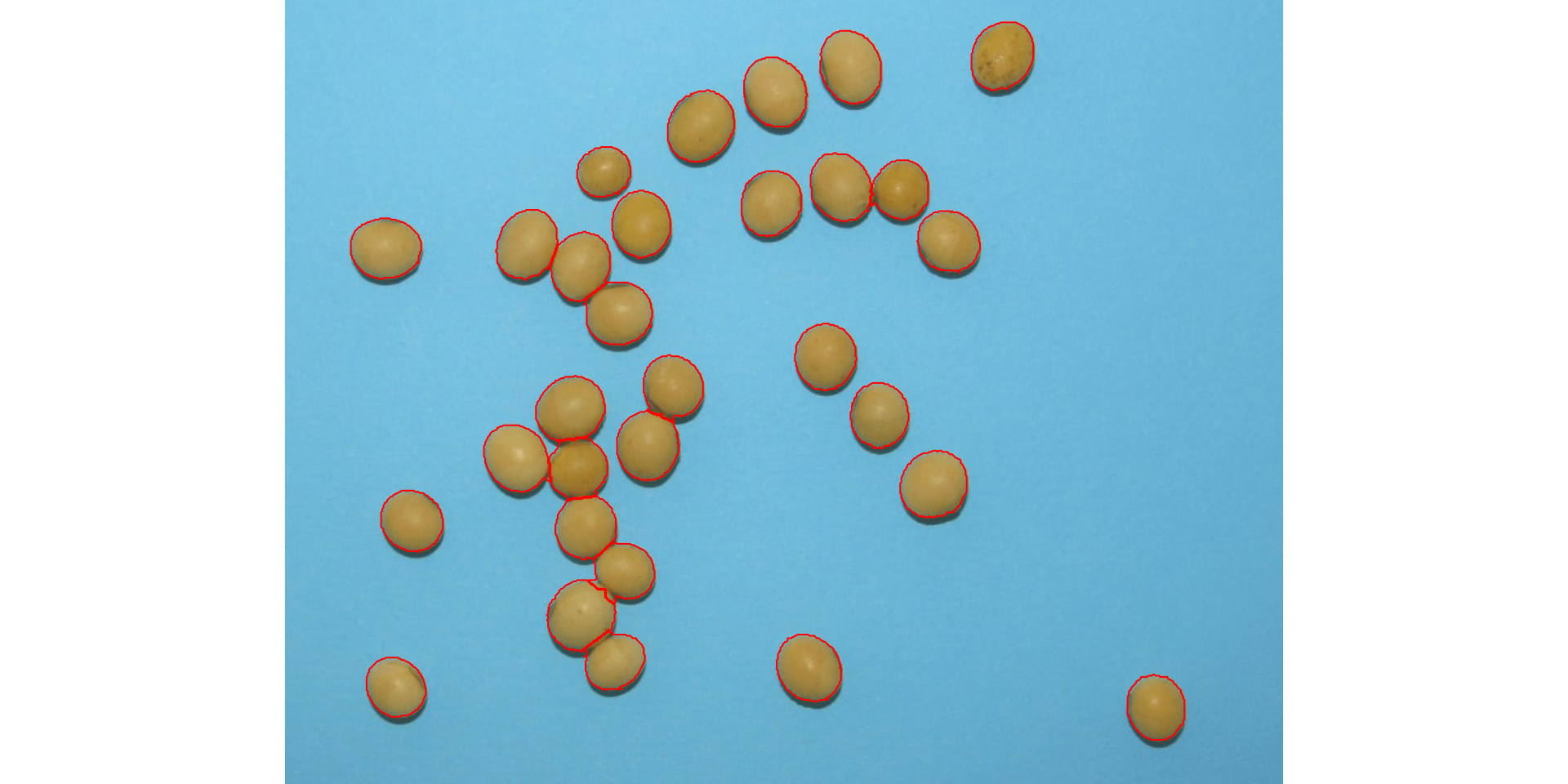Provided that the images are stored in the current working directory (or subdirectory), users can count the objects with no need to first import the image into the R environment. In this case, image names need to be declared as characters. Assuming that soy, background, and grain are the images saved into the current working directory, the same result as above is obtained with

analyze_objects(img = "soy",
background = "background",
foreground = "grain")

## Leaf shape

The function analyze_objects() computes a range of object features that can be used to study leaf shape. As a motivating example, I will use the image potato_leaves.png, which was gathered from Gupta et al. (2020)1

potato <- image_pliman("potato_leaves.jpg", plot = TRUE)
pot_meas <-
analyze_objects(potato,
watershed = FALSE,
marker = "id",
show_chull = TRUE) # shows the convex hull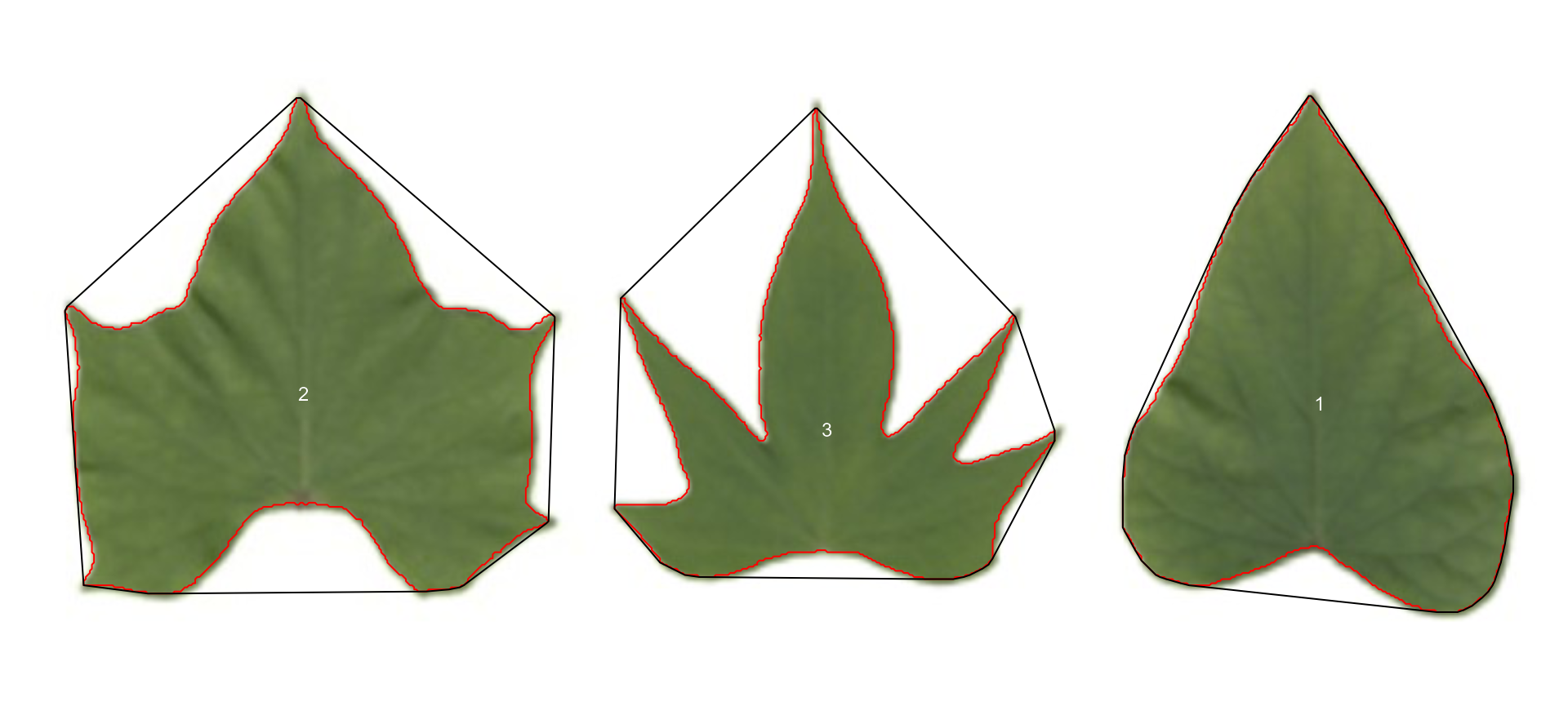str(pot_meas)
#> List of 14
#>  $results :'data.frame': 3 obs. of 34 variables: #> ..$ id                  : num [1:3] 1 2 3
#>   ..$x : num [1:3] 854 197 535 #> ..$ y                   : num [1:3] 223 217 240
#>   ..$area : num [1:3] 51398 58909 35177 #> ..$ area_ch             : num [1:3] 54600 76590 63138
#>   ..$perimeter : num [1:3] 1012 1255 1528 #> ..$ radius_mean         : num [1:3] 131 140 110
#>   ..$radius_min : num [1:3] 92 68.8 37.3 #> ..$ radius_max          : num [1:3] 199 193 189
#>   ..$radius_sd : num [1:3] 26.2 28.6 35.6 #> ..$ diam_mean           : num [1:3] 263 280 220
#>   ..$diam_min : num [1:3] 184 137.6 74.7 #> ..$ diam_max            : num [1:3] 398 385 378
#>   ..$major_axis : num [1:3] 105.4 106.8 83.6 #> ..$ minor_axis          : num [1:3] 82.9 95.2 80.1
#>   ..$caliper : num [1:3] 346 350 318 #> ..$ length              : num [1:3] 346 330 294
#>   ..$width : num [1:3] 255 331 309 #> ..$ radius_ratio        : num [1:3] 2.16 2.8 5.06
#>   ..$theta : num [1:3] 1.432 -0.167 0.505 #> ..$ eccentricity        : num [1:3] 0.617 0.454 0.287
#>   ..$form_factor : num [1:3] 0.631 0.47 0.189 #> ..$ narrow_factor       : num [1:3] 1 1.06 1.08
#>   ..$asp_ratio : num [1:3] 1.358 0.995 0.95 #> ..$ rectangularity      : num [1:3] 1.72 1.85 2.58
#>   ..$pd_ratio : num [1:3] 2.92 3.59 4.81 #> ..$ plw_ratio           : num [1:3] 1.68 1.9 2.54
#>   ..$solidity : num [1:3] 0.941 0.769 0.557 #> ..$ convexity           : num [1:3] 0.907 0.735 0.557
#>   ..$elongation : num [1:3] 0.2635 -0.0046 -0.0521 #> ..$ circularity         : num [1:3] 19.9 26.7 66.4
#>   ..$circularity_haralick: num [1:3] 5.02 4.9 3.1 #> ..$ circularity_norm    : num [1:3] 0.626 0.466 0.186
#>   ..$coverage : num [1:3] 0.1339 0.1534 0.0916 #>$ statistics      :'data.frame':    7 obs. of  2 variables:
#>   ..$stat : chr [1:7] "n" "min_area" "mean_area" "max_area" ... #> ..$ value: num [1:7] 3 35177 48495 58909 12129 ...
#>  $object_rgb : NULL #>$ object_index    : NULL
#>  $efourier : NULL #>$ efourier_norm   : NULL
#>  $efourier_error : NULL #>$ efourier_power  : NULL
#>  $efourier_minharm: NULL #>$ veins           : NULL
#>  $angles : NULL #>$ mask            : NULL
#>  $pcv : NULL #>$ parms           :List of 1
#>   ..\$ index: chr "NB"
#>  - attr(*, "class")= chr "anal_obj"

Three key measures (in pixel units) are:

1. area the area of the object.
2. area_ch the area of the convex hull.
3. perimeter the perimeter of the object.

Using these measures, circularity and solidity are computed as shown in (Gupta et al, 2020).

$circularity = 4\pi(area / perimeter^2)$

$solidity = area / area\_ch$

Circularity is influenced by serrations and lobing. Solidity is sensitive to leaves with deep lobes, or with a distinct petiole, and can be used to distinguish leaves lacking such structures. Unlike circularity, it is not very sensitive to serrations and minor lobings, since the convex hull remains largely unaffected.

### Object contour

Users can also obtain the object contour and convex hull as follows:

cont <-
object_contour(potato,
watershed = FALSE,
plot = FALSE)
plot(potato)
plot_contour(cont, col = "red", lwd = 3)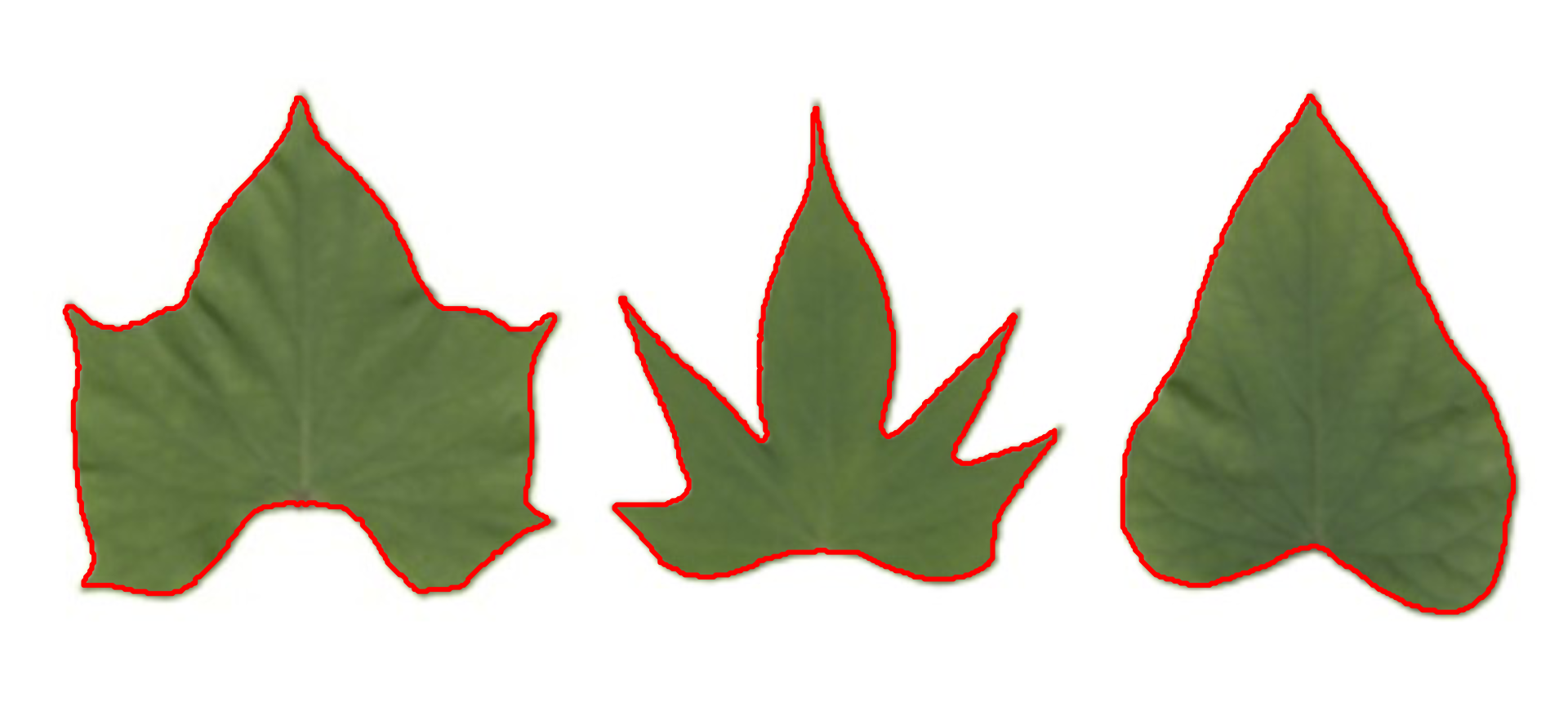### Convex hull

The function object_contour() returns a list with the coordinate points for each object contour that can be further used to obtain the convex hull with conv_hull().

conv <- conv_hull(cont)
plot(potato)
plot_contour(conv, col = "red", lwd = 3)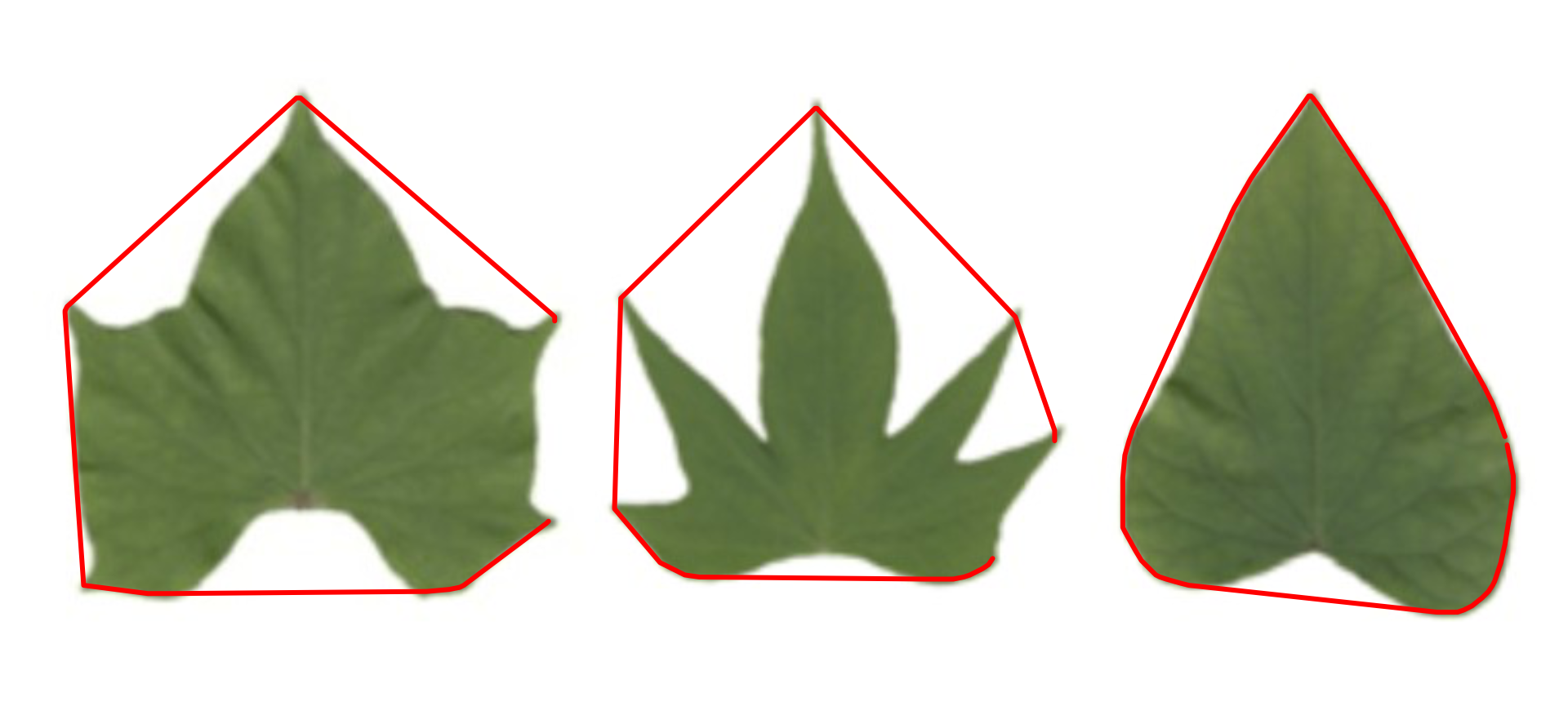### Area of the convex hull

Then, the area of the convex hull can be obtained with poly_area().

(area <- poly_area(conv))
#>  54600.0 76589.5 63138.0

### Leaves as base plot

# create a data frame for contour and convex hull
df_cont <-
do.call(rbind,
lapply(seq_along(cont), function(i){
transform(as.data.frame(cont[[i]]), object = names(cont[i]))
}))

df_conv <-
do.call(rbind,
lapply(seq_along(conv), function(i){
transform(as.data.frame(conv[[i]]), object = names(conv[i]))
}))

# plot the objects
palette(c("red","blue","green"))
with(df_cont,
plot(V1, V2,
cex = 0.5,
col = object,
xlab = NA,
ylab = NA,
axes = F))
with(subset(df_conv, object == 1),
polygon(V1, V2,
col = rgb(1, 0, 0, 0.2),
border = NA))
with(subset(df_conv, object == 2),
polygon(V1, V2,
col = rgb(0, 0, 1, 0.2),
border = NA))
with(subset(df_conv, object == 3),
polygon(V1, V2,
col = rgb(0, 1, 0, 0.2),
border = NA))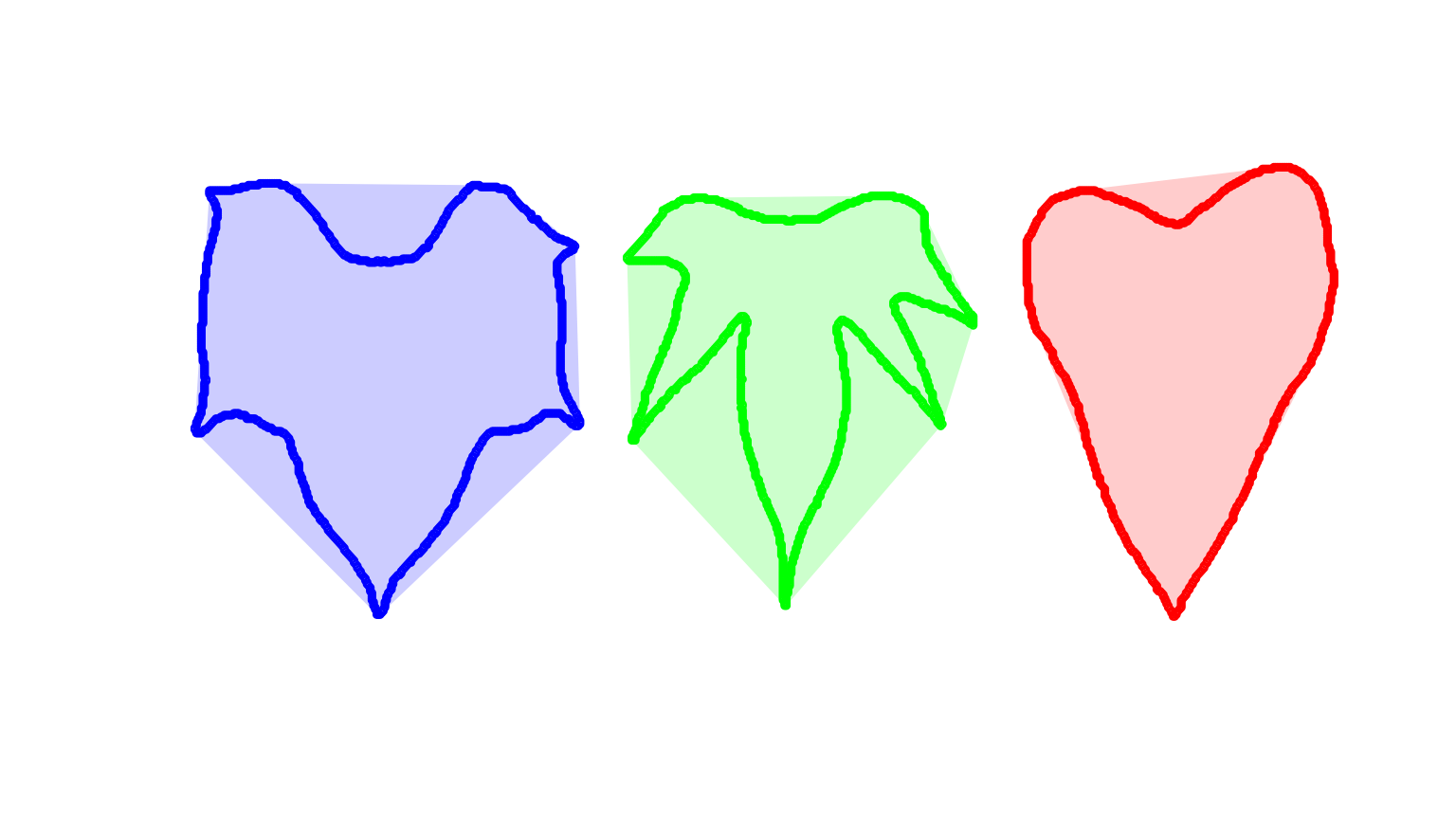Or do the same with ggplot2

library(ggplot2)
ggplot(df_cont, aes(V1, V2, group = object)) +
geom_polygon(aes(fill = object)) +
geom_polygon(data = df_conv,
aes(V1, V2, fill = object),
alpha = 0.3) +
theme_void() +
theme(legend.position = "bottom")

## Batch processing

In plant image analysis, frequently it is necessary to process more than one image. For example, in plant breeding, the number of grains per plant (e.g., wheat) is frequently used in the indirect selection of high-yielding plants. In pliman, batch processing can be done when the user declares the argument pattern.

The following example would be used to count the objects in the images with a pattern name "trat" (e.g., "trat1", "trat2", "tratn") saved into the subfolder “originals" in the current working directory. The processed images will be saved into the subfolder "processed". The object list_res will be a list with two objects (results and statistics) for each image.

To speed up the processing time, especially for a large number of images, the argument parallel = TRUE can be used. In this case, the images are processed asynchronously (in parallel) in separate R sessions running in the background on the same machine. The number of sections is set up to 50% of available cores. This number can be controlled explicitly with the argument workers.

list_res <-
analyze_objects(pattern = "trat", # matches the name pattern in 'originals' subfolder
dir_original = "originals",
dir_processed = "processed",
parallel = TRUE, # parallel processing
workers = 8, # 8 multiple sections
save_image = TRUE)

## A little bit more!

At this link you will find more examples on how to use {pliman} to analyze plant images. Source code and images can be downloaded here. You can also find a talk (Portuguese language) about {pliman} here. Lights, camera, {pliman}!